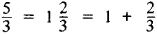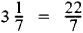# improper fraction

Also found in: Dictionary, Thesaurus, Wikipedia.

## improper fraction

a fraction in which the numerator has a greater absolute value or degree than the denominator, as 7/6 or (x2 + 3)/(x + 1)
Collins Discovery Encyclopedia, 1st edition © HarperCollins Publishers 2005
The following article is from The Great Soviet Encyclopedia (1979). It might be outdated or ideologically biased.

## Improper Fraction

an arithmetic fraction whose numerator is greater than or equal to the denominator—for example, 5/3, 4/2, and 7/7. An improper fraction can be represented, by separating its integral part, in the form of a mixed number—that is, a number having an integral part and a fractional part, for example,Conversely, every mixed number can be written in the form of an improper fraction, for example,## improper fraction

[im′präp·ər ′frak·shən]
(mathematics)
In arithmetic, the quotient of two integers in which the numerator is greater than or equal to the denominator.
In algebra, the quotient of two polynomials in which the degree of the numerator is greater than or equal to that of the denominator.
McGraw-Hill Dictionary of Scientific & Technical Terms, 6E, Copyright © 2003 by The McGraw-Hill Companies, Inc.
Mentioned in ?
References in periodicals archive ?
Step 4: The result 4 is an improper fraction and must be reduced to a mixed number, which would be 1 1/4.
6, an equation 1 25 = 13/5 can be recognized as an error step of converting a mixed number to an improper fraction. Similarly, an equation 1 3/5 = 4/5 also can be recognized as an error step with the aid of Eq.
Students, who have only learned the shortcut algorithm for renaming mixed numbers as improper fractions, may not know that a mixed number is a sum.
The data from 323 interviews with students at the end of Year 6 (Clarke, Roche, Mitchell & Sukenik, 2006) indicated that students need classroom experiences which assist them to understand more clearly the roles of the numerator and denominator in a fraction, the meaning of improper fractions, and the relative sizes of fractions.
For example, an environment for solving linear equations can accept both decimal and common fractions or mixed numbers and improper fractions as answers.
Grade 4 Introduction of fractions; improper fractions and mixed numbers; comparison of fractions (with like denominators only) Grade 5 Comparison of fractions (unlike denominators); equivalent fractions; addition and subtraction of fractions with like denominators; fractions as quotient; relationships among fractions, decimals & whole numbers Grade 6 Addition and subtraction of fractions with unlike denominators; creating equivalent fractions; multiplication and division of fractions Table 2.
These operations suggest that Joe was developing a scheme for generating improper fractions.
For example, it could be argued that the number line is better suited to representing improper fractions than either the area or set models.
For example, in one classroom, the teacher used the Interactive Whiteboard to illustrate how to use simple regional models to explore improper fractions. The teacher gave the students a fraction (such as 5/3) and the students illustrated 5/3 using circle pieces (3 1/3rds to make one whole plus 2 additional thirds).
Varying the numbers in these problems produces a variety of fractions as solutions, including unit fractions, such as 1/3 for three children sharing one candy bar; nonunit fractions, such as 3/4 in the candy-bar problem; improper fractions, such as 10/4 for four children sharing ten candy bars; and mixed numbers, such as 2 1/6 for six children sharing thirteen candy bars.
Over the course of the intervention, we gradually introduced more complicated fractions, equivalent fractions, improper fractions and a wider breadth of representations of fractions.
When one student named a fraction as "fifteen ninths", his partner demonstrated that the fifteen pieces on the twenty-four mat equaled fifteen twenty-fourths, saying, "But we have fifteen pieces and it takes twenty-four to make a whole." This came about because the author previously reviewed that the denominator meant "the number of pieces it takes to make a whole", rather than "the number of pieces in all" to clarify confusion and allow the definition to include improper fractions.

Site: Follow: Share:
Open / Close